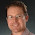## Friday, December 04, 2009

### Greatest Common Divisor

Euclid's algorithm for computing the greatest common divisor of two integers is beautiful because it is extremely simple and also captures an interesting property of linear equations. The equation ax+by = c has an integer solution if and only if gcd(a,b) divides c, where gcd is Euclid's algorithm written below. Recall that x divides y means there exists some n such that xn = y.
gcd(a,b) =  if a == 0 then     b  else if b == 0 then     a  else if b < a then     gcd(a - b, b)  else     gcd(a, b - a)
Proving that Euclid's algorithm really works is a good exercise in applying strong induction. We are going to prove that gcd(a,b) is the greatest common divisor of a and b. To apply strong induction, we need to pick a number to do induction on. The numbers a or b are obvious candidates, but neither does the job. Consider the two branches of the "if" expression in gcd. If we choose to do induction on a, then the "else" branch will cause us trouble because we won't be able to apply the induction hypothesis for gcd(a, b - a). If we choose to do induction on b, then we'll have the same kind of trouble in the "then" branch. We need some number that gets smaller in both branches. It turns out that a + b is such a number.

Theorem (Correctness of gcd).
gcd(a,b) is the greatest common divisor of a and b.
Proof.
We proceed by strong induction on a + b. When trying to prove something about a function like gcd, it often helps to structure your proof in a way that mimics the definition of the function. That is, we'll do case analysis in the proof in a way that matches the cases in the definition of gcd.
Case a = 0:
In this case gcd(a,b) = b. We know that b divides 0 and b divides b. Also, for any other divisor d of a and b, it is trivially true that d divides b. Thus, gcd(a,b) is the greatest common divisor of a and b.
Case not (a = 0) and b = 0:
The reasoning is the mirror image of the previous case and left for the reader.
Case not (a = 0) and not (b = 0):
Without loss of generality, assume that b < a. Then gcd(a,b) = gcd(a - b, b). Note that (a - b) + b < a + b. So by the induction hypothesis we know that gcd(a - b, b) is the greatest common divisor of a - b and b and so is its equal, gcd(a,b). Because gcd(a,b) divides both a - b and b, gcd(a,b) divides a, so gcd(a,b) is a common divisor of a and b. To finish we need to show it is the greatest. Assume d is an arbitrary common divisor of a and b. Then d divides a - b and because gcd(a,b) is the greatest common divisor of a - b and b, we can conclude that d divides gcd(a,b). We therefore proved that gcd(a,b) is the greatest common divisor of a and b.
QED.

A proof of this theorem in Isabelle can be found here.

#### 2 comments:

1.Sorry, I find greatest part of GCD is not very clear to me.

Not an expert on Isabelle, but your proof seems take this as a fact, rather than proof it. (by using greatest_common_divisor_def) Could you comment on that?

1.The use of greatest_common_divisor_def merely unfolds the definition of what it means to be the greatest common divisor, which is given at the top of the Isabelle file. The whole sub-proof labeled 'greatest' is the reasoning that proves it is the greatest. Note that by greatest we don't literally mean the largest. The ordering is in terms of the divides relation.